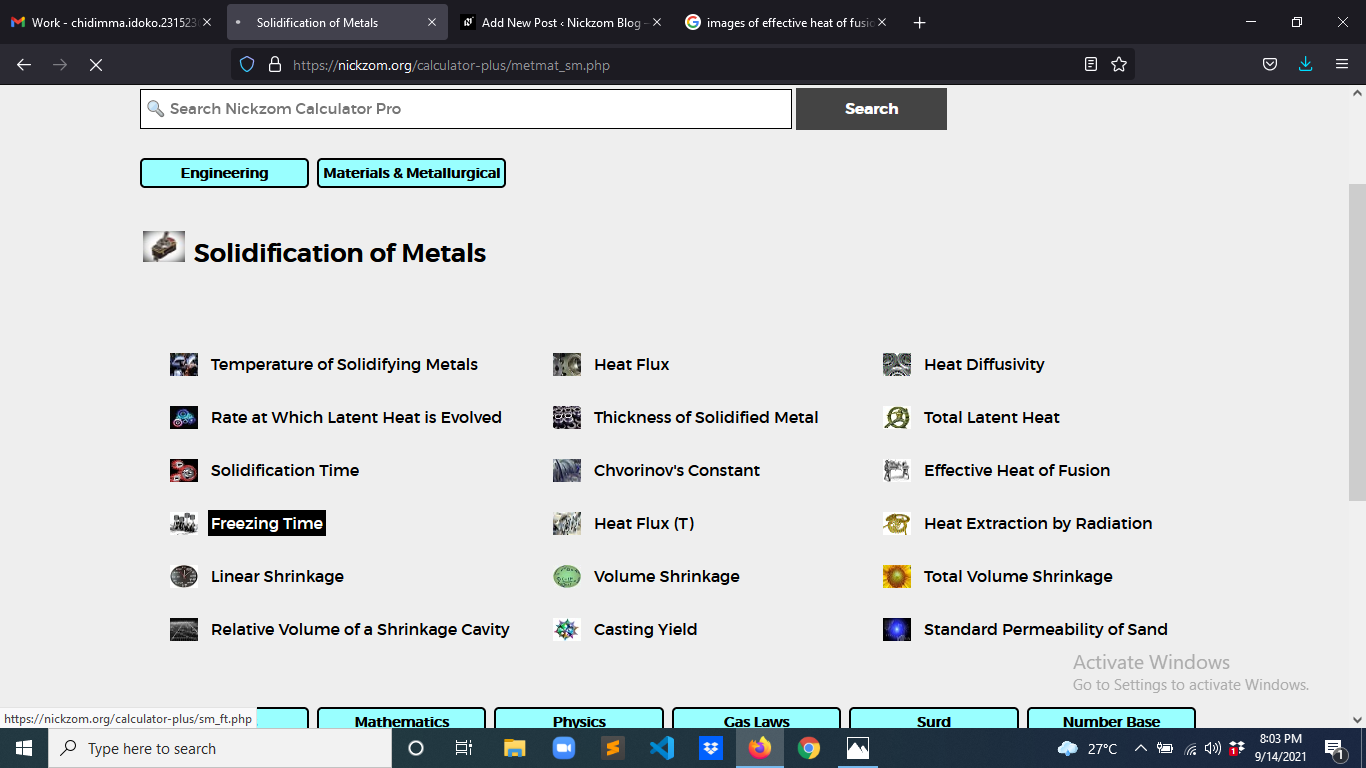# How to Calculate and Solve for Freezing Time | Solidification of MetalsThe image above represents freezing time.

To compute for freezing time, three essential parameters are needed and these parameters are Thickness of Solidified Metal (M), Constant (β) and Heat Diffusivity (α).

The formula for calculating freezing time:

t = / 4β²α

Where:

t = Freezing Time
M = Thickness of Solidified Metal
β = Constant
α = Heat Diffusivity

Let’s solve an example;
Find the freezing time when the thickness of solidified metal is 8, the constant is 10 and the heat diffusivity is 2.

This implies that;

M = Thickness of Solidified Metal = 8
β = Constant = 10
α = Heat Diffusivity = 2

t = / 4β²α
t = / 4(10)²(2)
t = 64 / 4(100)(2)
t = 64 / 800
t = 0.08

Therefore, the freezing time is 0.08 s.

Calculating the Thickness of Solidified Metal when the Freezing Time, the Constant and the Heat Diffusivity is Given.

M = t x 4β²α

Where:

M = Thickness of Solidified Metal
t = Freezing Time
β = Constant
α = Heat Diffusivity

Let’s solve an example;
Find the thickness of solidified metal when the freezing time is 21, the constant is 9 and the heat diffusivity is 2.

This implies that;

t = Freezing Time = 21
β = Constant = 9
α = Heat Diffusivity = 2

M = t x 4β²α
M = 21 x 4(9²)(2)
M = 21 x 4(81)(2)
M = 21 x 4(162)
M = 21 x 648
M = 13668

Therefore, the thickness of solidified metal is 13668.

Calculating the Constant when the Freezing Time, the Thickness of Solidified Metal and the Heat Diffusivity is Given.

β = √(M / t x 4α)

Where:

β = Constant
t = Freezing Time
M = Thickness of Solidified Metal
α = Heat Diffusivity

Let’s solve an example;
Find the constant when the freezing time is 4, the thickness of solidified metal is 40 and the heat diffusivity is 2.

This implies that;

t = Freezing Time = 4
M = Thickness of Solidified Metal = 40
α = Heat Diffusivity = 2

β = √(40 / 4 x 4(2))
β = √(40 / 4 x 8)
β = √(40 / 32)
β = √(1.25)
β = 1.11

Therefore, the constant is 1.11.

Calculating the Heat Diffusivity when the Freezing Time, the Thickness of Solidified Metal and the Constant is Given.

α = M / t x 4β²

Where:

α = Heat Diffusivity
t = Freezing Time
M = Thickness of Solidified Metal
β = Constant

Let’s solve an example;
Given that the freezing time is 2, the thickness of solidified metal is 30 and the constant is 1. Find the heat diffusivity?

This implies that;

t = Freezing Time = 2
M = Thickness of Solidified Metal = 30
β = Constant = 1

α = M / t x 4β²
α = 30 / 2 x 4(1²)
α = 30 / 8
α = 3.75

Therefore, the heat diffusivity is 3.75.

Nickzom Calculator – The Calculator Encyclopedia is capable of calculating the freezing time.

To get the answer and workings of the freezing time using the Nickzom Calculator – The Calculator Encyclopedia. First, you need to obtain the app.

You can get this app via any of these means:

To get access to the professional version via web, you need to register and subscribe for NGN 2,000 per annum to have utter access to all functionalities.
You can also try the demo version via https://www.nickzom.org/calculator

Apple (Paid) – https://itunes.apple.com/us/app/nickzom-calculator/id1331162702?mt=8
Once, you have obtained the calculator encyclopedia app, proceed to the Calculator Map, then click on Materials and Metallurgical under Engineering.Now, Click on Solidification of Metal under Materials and MetallurgicalNow, Click on Freezing Time under Solidification of MetalThe screenshot below displays the page or activity to enter your values, to get the answer for the freezing time according to the respective parameter which is the Thickness of Solidified Metal (M), Constant (β) and Heat Diffusivity (α).Now, enter the values appropriately and accordingly for the parameters as required by the Thickness of Solidified Metal (M) is 8, Constant (β) is 10 and Heat Diffusivity (α) is 2.Finally, Click on CalculateAs you can see from the screenshot above, Nickzom Calculator– The Calculator Encyclopedia solves for the freezing time and presents the formula, workings and steps too.